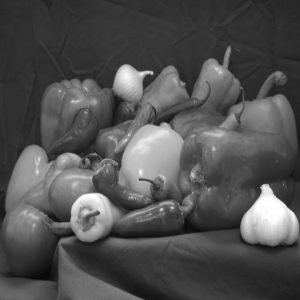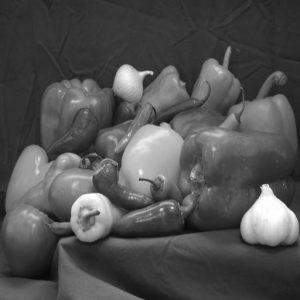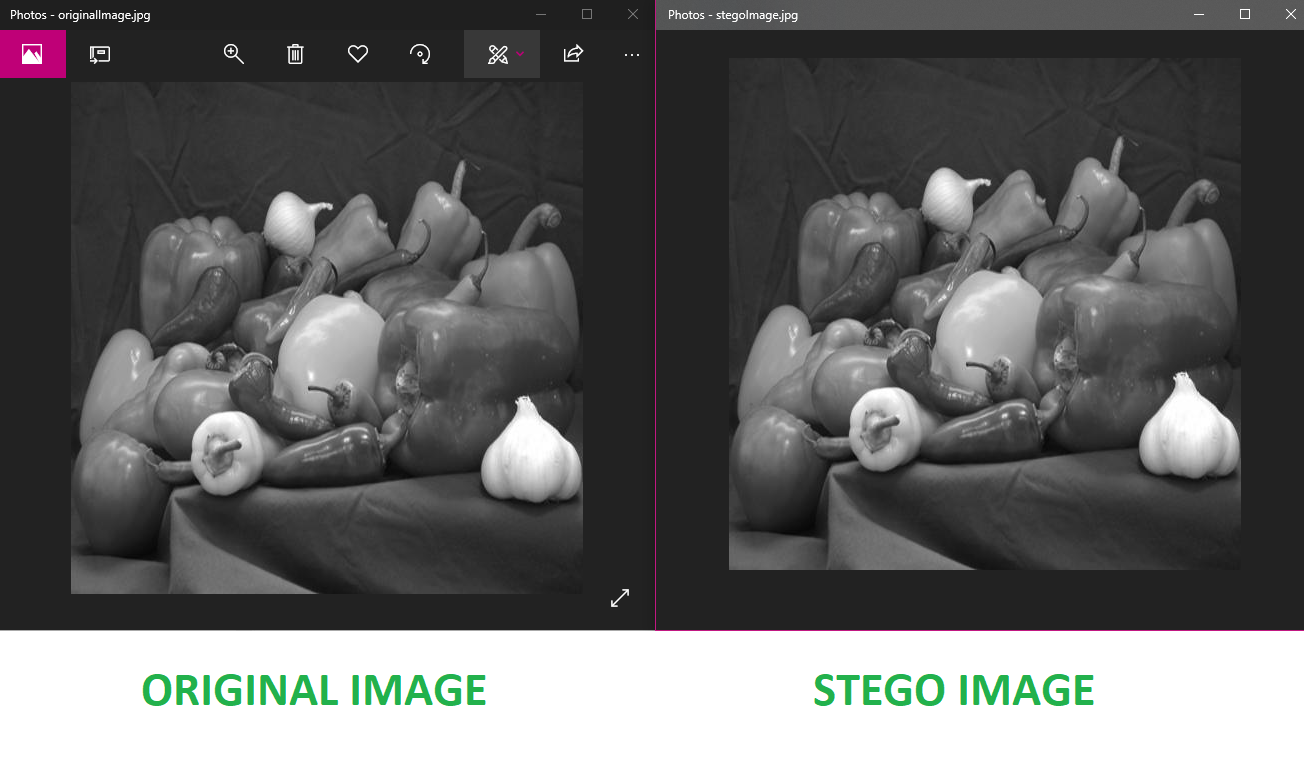Open in App
Not now

# LSB based Image steganography using MATLAB

• Difficulty Level : Basic
• Last Updated : 12 Dec, 2021

Prerequisite: Image Steganography Introduction, Implementation using Python.
Steganography is the method of hiding secret data inside any form of digital media. The main idea behind steganography is to hide the existence of data in any medium like audio, video, image, etc. When we talk about image steganography, the idea is quite simple. Images are made up of pixels which usually refer to the color of that particular pixel. In a grayscale (black and white) image, these pixel values range from 0-255, 0 being black and 255 being white.
Concept of LSB based data embedding:
LSB stands for Least Significant Bit. The idea behind LSB embedding is that if we change the last bit value of a pixel, there won’t be much visible change in the color. For example, 0 is black. Changing the value to 1 won’t make much of a difference since it is still black, just a lighter shade.
The encoding is done using the following steps:

1. Convert the image to grayscale
2. Resize the image if needed
3. Convert the message to its binary format
4. Initialize output image same as input image
5. Traverse through each pixel of the image and do the following:
• Convert the pixel value to binary
• Get the next bit of the message to be embedded
• Create a variable temp
• If the message bit and the LSB of the pixel are same, set temp = 0
• If the message bit and the LSB of the pixel are different, set temp = 1
• This setting of temp can be done by taking XOR of message bit and the LSB of the pixel
• Update the pixel of output image to input image pixel value + temp
6. Keep updating the output image till all the bits in the message are embedded
7. Finally, write the input as well as the output image to local system.

Example:
Input : message=’geeksforgeeks’Output : Image with the given message embedded:Below is the implementation in MATLAB:

## matlab

 `% Clear the existing workspace` `clear all;`   `% Clear the command window` `clc;`   `% Read the input image` `input = imread(``'peppers.png'``);`   `% Convert image to grayscale` `input=rgb2gray(input);`   `% Resize the image to required size` `input=imresize(input, [512 512]);`   `% Message to be embedded` `message=``'geeksforgeeks'``;`   `% Length of the message where each character is 8 bits` `len = length(message) * 8;`   `% Get all the ASCII values of the characters of the message` `ascii_value = uint8(message);`   `% Convert the decimal values to binary` `bin_message = transpose(dec2bin(ascii_value, 8));`   `% Get all the binary digits in separate row` `bin_message = bin_message(:);`   `% Length of the binary message` `N = length(bin_message);`   `% Converting the char array to numeric array` `bin_num_message=str2num(bin_message);`   `% Initialize output as input` `output = input;`   `% Get height and width for traversing through the image` `height = size(input, 1);` `width = size(input, 2);`   `% Counter for number of embedded bits` `embed_counter = 1;`   `% Traverse through the image` `for` `i = 1 : height` `    ``for` `j = 1 : width` `        `  `        ``% If more bits are remaining to embed` `        ``if``(embed_counter <= len)` `            `  `            ``% Finding the Least Significant Bit of the current pixel` `            ``LSB = mod(double(input(i, j)), 2);` `            `  `            ``% Find whether the bit is same or needs to change` `            ``temp = double(xor(LSB, bin_num_message(embed_counter)));` `            `  `            ``% Updating the output to input + temp` `            ``output(i, j) = input(i, j)+temp;` `            `  `            ``% Increment the embed counter` `            ``embed_counter = embed_counter+1;` `        ``end` `        `  `    ``end` `end`   `% Write both the input and output images to local storage` `% Mention the path to a folder here.` `imwrite(input, ``'path_to_folder\originalImage.png'``);` `imwrite(output, ``'path_to_folder\stegoImage.png'``);`

Comparison of images:As we can see in the above screenshot, the input and the output image look exactly the same to the human eye. The output image has the message embedded in it.

• This method is very fast and easy to implement in comparison to other methods of image Steganography.
• The output image has very slight difference to the input image.
• Instead of embedding the message in only the LSB, we can embed the message in last two LSBs, thus embedding even large messages.
• This method forms the basics of many other complex algorithms
• Instead of embedding the message in only the LSB, we can embed the message in last two LSBs, thus embedding even large messages.

• This type of encoding the data is weak since it can be easily decoded by taking the LSBs of the image and getting the message in binary format.
• This is method is too old because it was used long ago when other encoding methods were not yet developed.
• When embedding the message in more than one LSB, the image quality may reduce depending on how many pixels are changed.

My Personal Notes arrow_drop_up
Related Articles